Home » Math » FREE Bright and Fun Task Cards To Multiply Decimals with Models

# FREE Bright and Fun Task Cards To Multiply Decimals with Models

Practice how to multiply decimals with models using these FREE task cards. Children will color in decimal grids to solve problems, as well as create equations based on a colored in a decimal grid.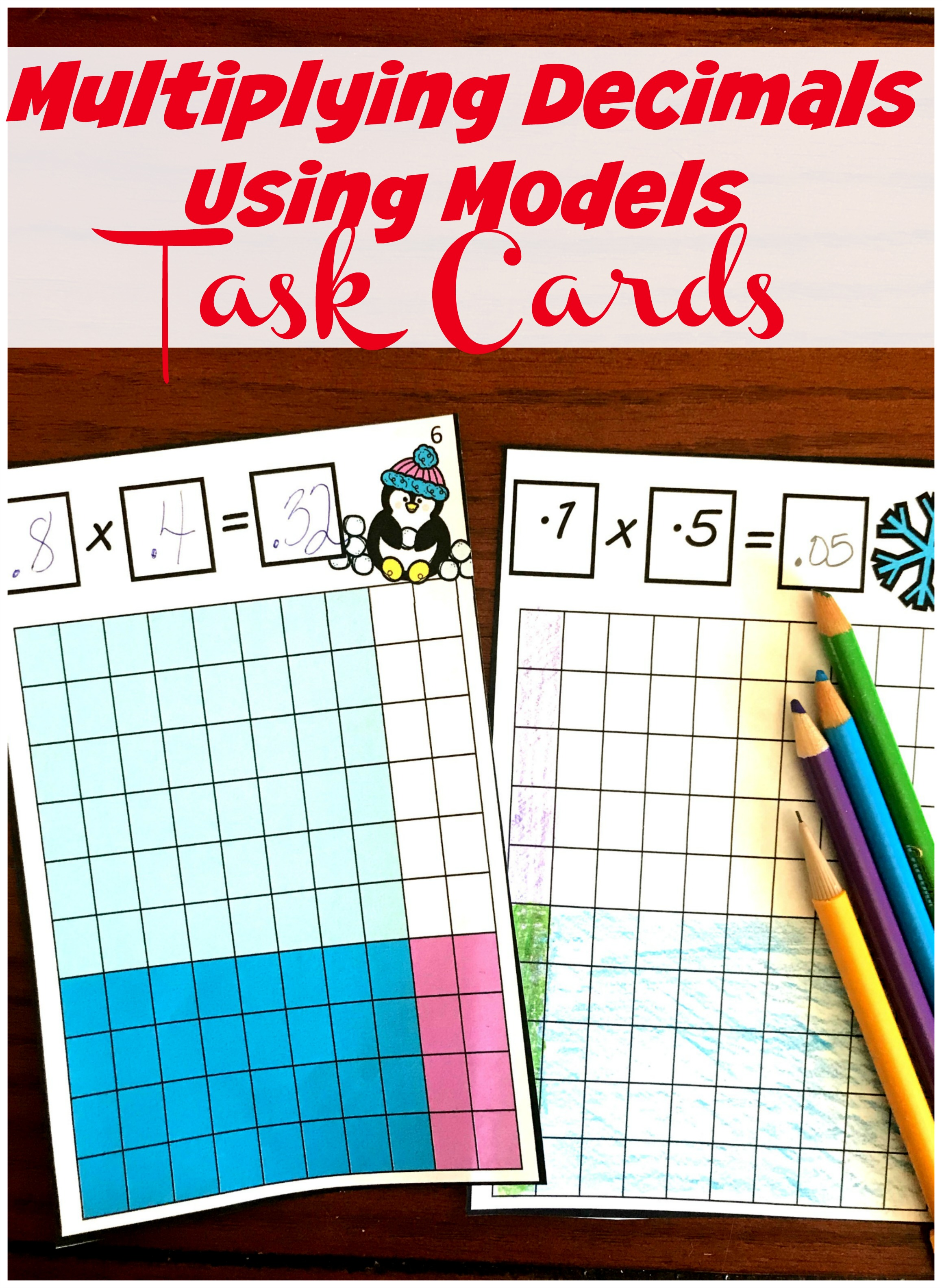You can get the FREE printable by clicking on the large blue button at the very end of the post.

If you have read any of my blog, you know I have a passion for math. But it isn’t just creating games to make math a little more fun, it is about helping children understand math. To not just be able to solve a problem with a formula, but to be able to explain why the formula works.

Area models are one easy way to do this. They can be used with multiplication, division, fractions, and even decimals. And today I have some FREE task cards to help your children multiply decimals with models.

## Multiply Decimals with Models

Now that the task cards are ready. How do we create models to find the answer to a multiplication problem involving decimals.

I’m glad you asked, and it is a lot easier than you think it is.

1. First, we begin by looking that the first factor and color that number in on the decimal grid.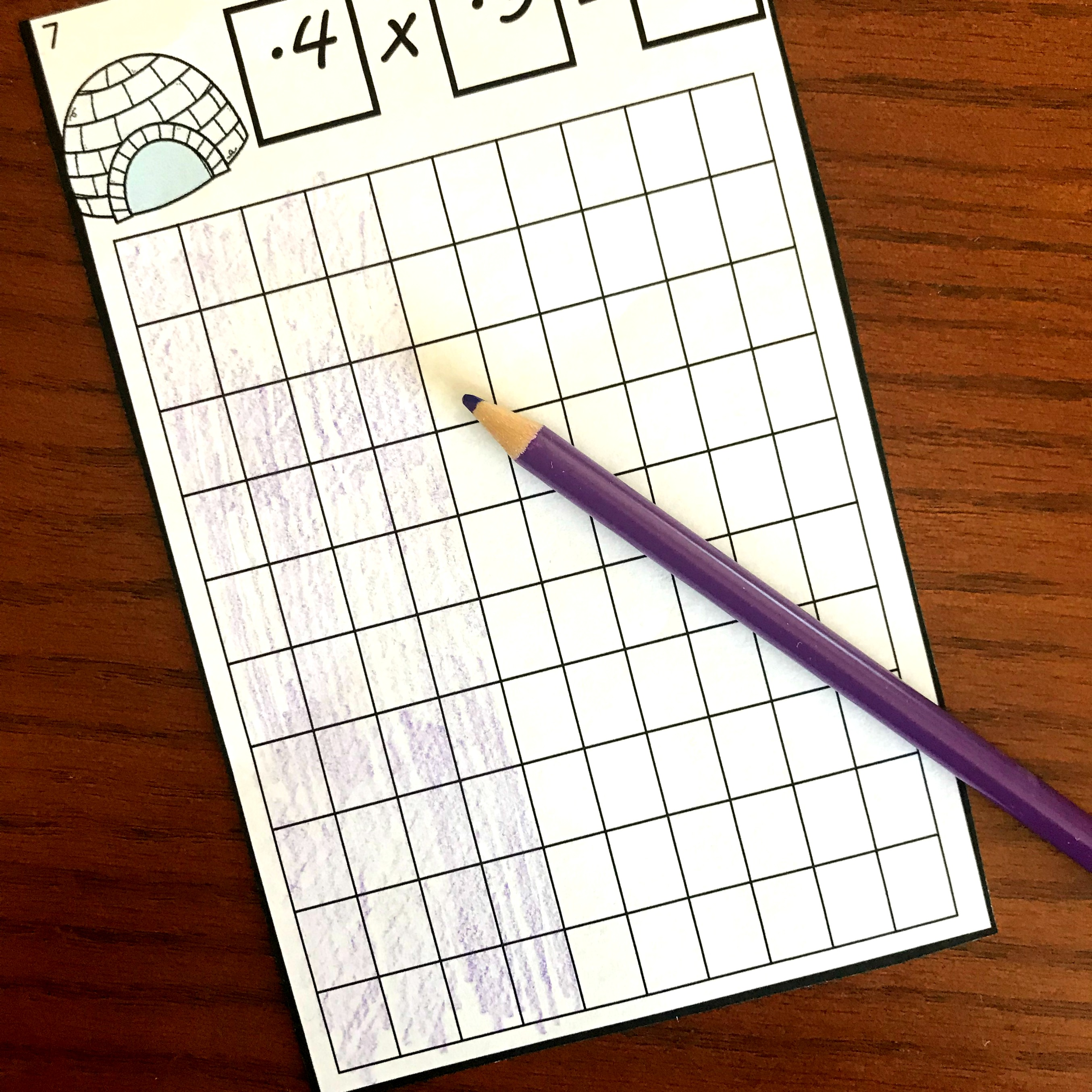The first factor is four tenths, so we color in four out of the 10 columns.

2. Next, we look at the second product and color in that number on the decimal grid. The only thing to be aware of here is to do the opposite of what you did last time. If you colored in the first factor horizontally, you will need to color in the second factor vertically.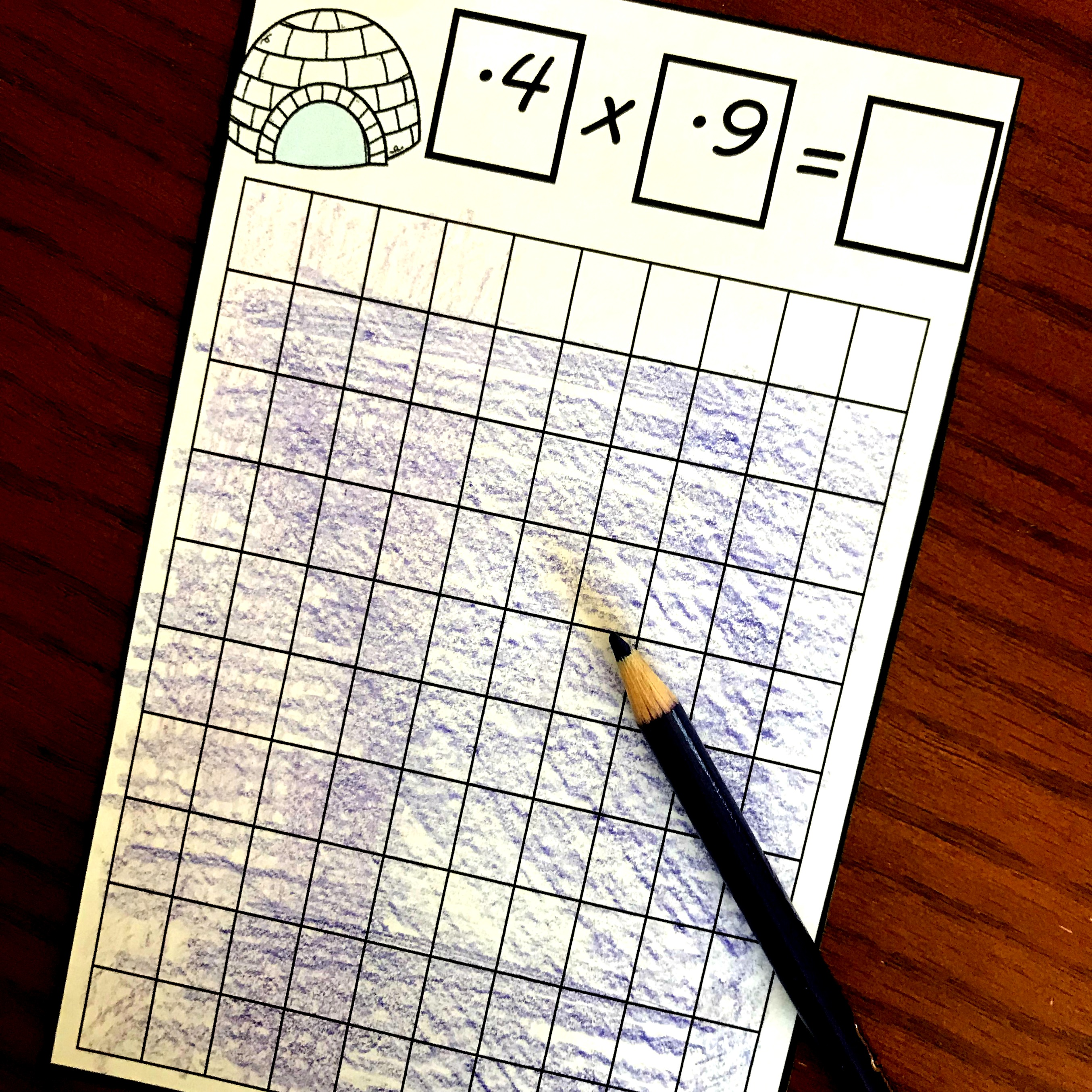The second factor is nine-tenths, so we color in nine out of the ten rows.

3. Finally,  it is time to count up how many of the squares are colored in twice. This is the answer.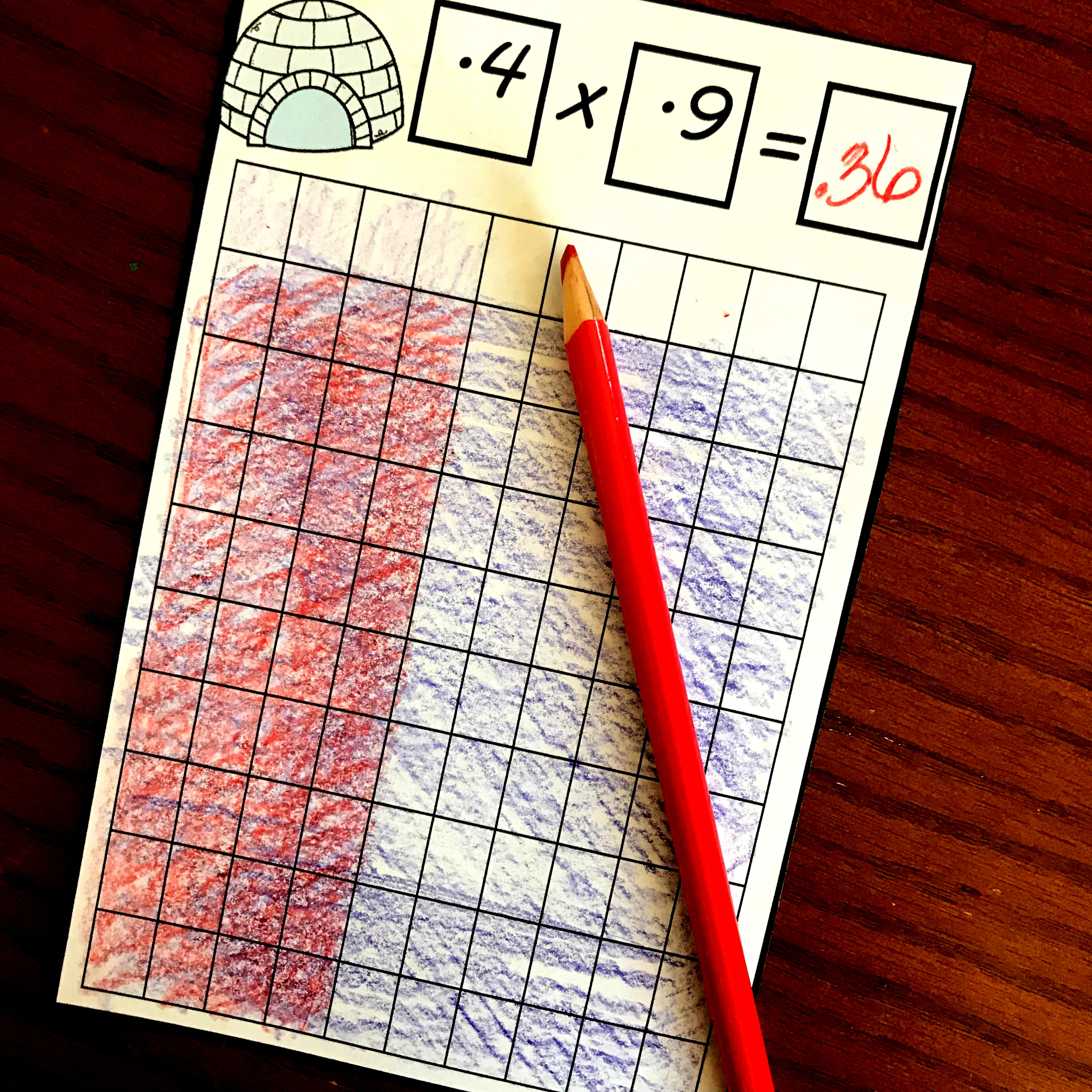There are 36 out of 100 squares that have both the purple and the blue color…..so the answer is .36 or thirty-six hundredths.

## Finding an Equation from the Decimal Grid

Some of the task cards have the model already colored in, and the children get to figure out the equation.

1. First, the look at one color and decide what decimal that color represents.

2. Next, they look at the second color and figure out what decimal that color represents. This will be the second factor.

3. Finally, they look at the color that shows where the two factors overlap. This is the product or answer.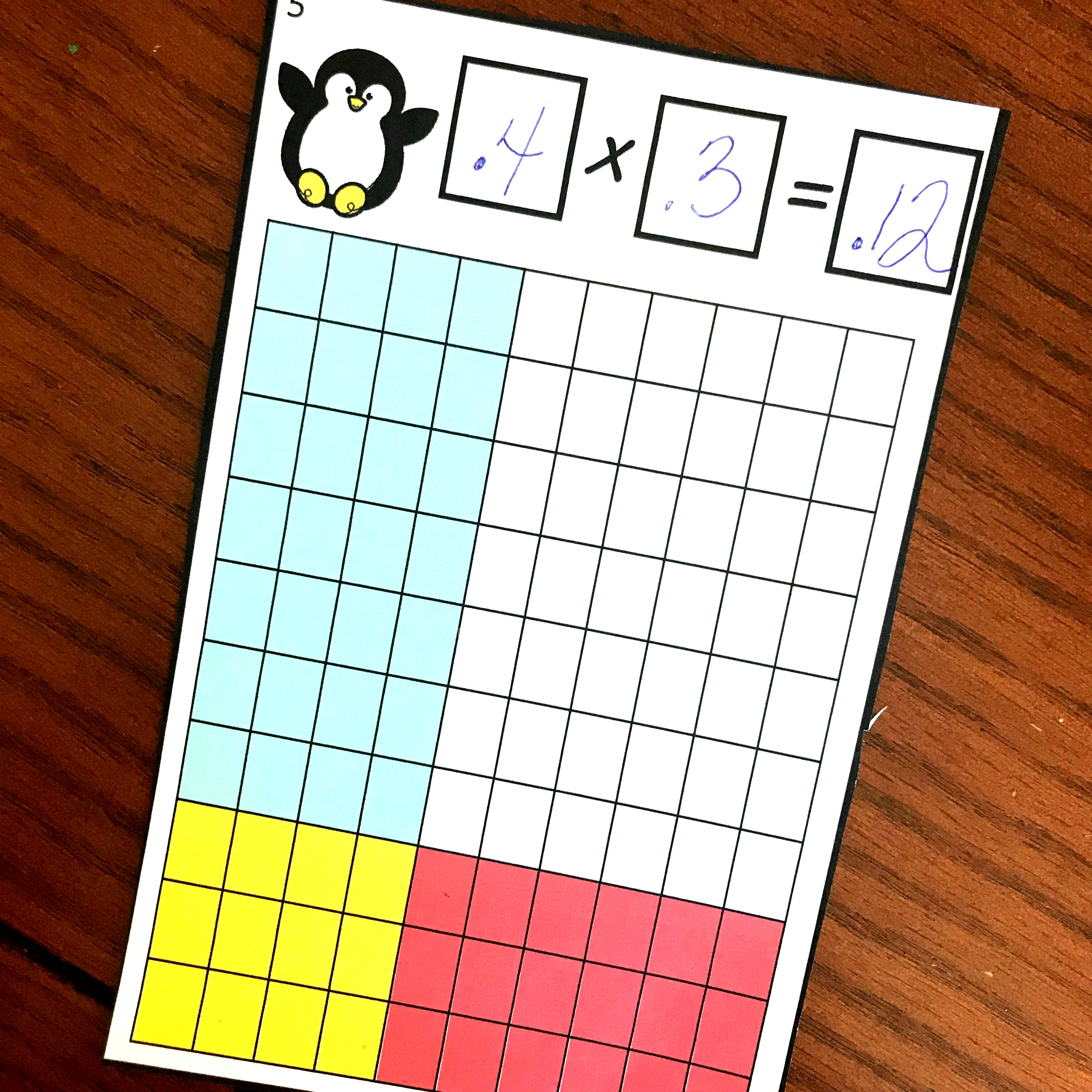There are three out of 10 columns colored in blue (0.4) and three out of 10 rows colored in red (0.3). This means that there are 12 out of 100 squares (0.12) that have been colored in twice. So my equation is .4 x .3 = .12

Having children Multiply Decimals with Models helps them understand why the answer will be smaller, and also help them understand where decimals go.

Enjoy!

You’ve Got This,

Rachel

You may also like:

Multiplying Decimals and Whole Numbers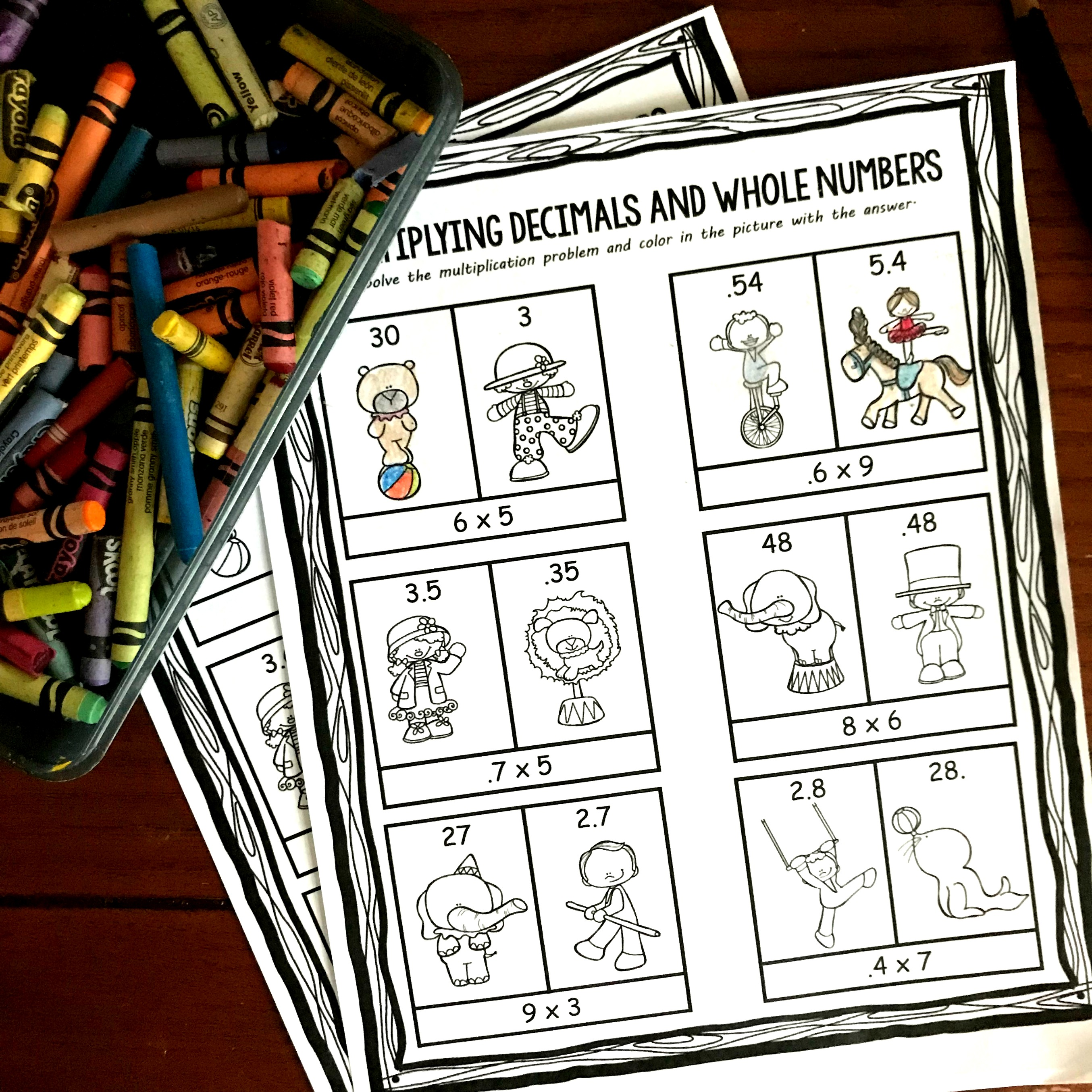Or one of my most popular bundles...Introducing Decimals. It will teach your students how to model decimals!This is a subscriber freebie. If you are already a subscriber, check the most recent email for the latest password. If you aren’t a subscriber, sign up below and the password will be provided in the first email you receive. You will also get \$2 off to use the You’ve Got This Store.

You’ll find it under the decimal section!